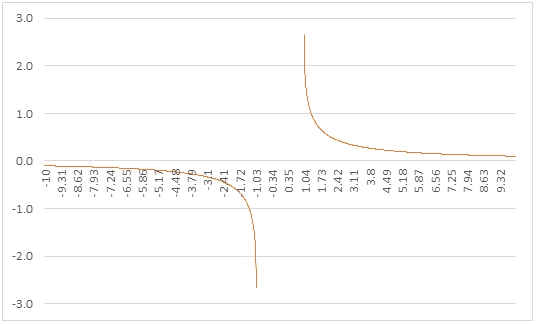# SQL Server ACOTH function

ACOTH
Updated: 24 Apr 2017

Use the scalar function ACOTH to calculate the hyperbolic arccotangent of a number.

Syntax
SELECT [wct].[ACOTH] (
<@Z, float,>)
Arguments
 Input Name Description @Z The cotangent of the angle. @Z must be of type float or of a type that implicitly converts to float.
Return Type
float
Remarks
Examples
Example #1
SELECT
wct.ACOTH(6) as ACOTH

This produces the following result.

Example #2

The following SQL can be run and the results pasted into Excel to graph the results of the function in the range -10, 10.

SELECT
seriesValue as z
,wct.ACOTH(Seriesvalue) as ACOTH
FROM
wct.SERIESFLOAT(-10,10,.01,NULL,NULL)

Here's the graph of the results.Copyright 2008-2023 Westclintech LLC         Privacy Policy        Terms of Service